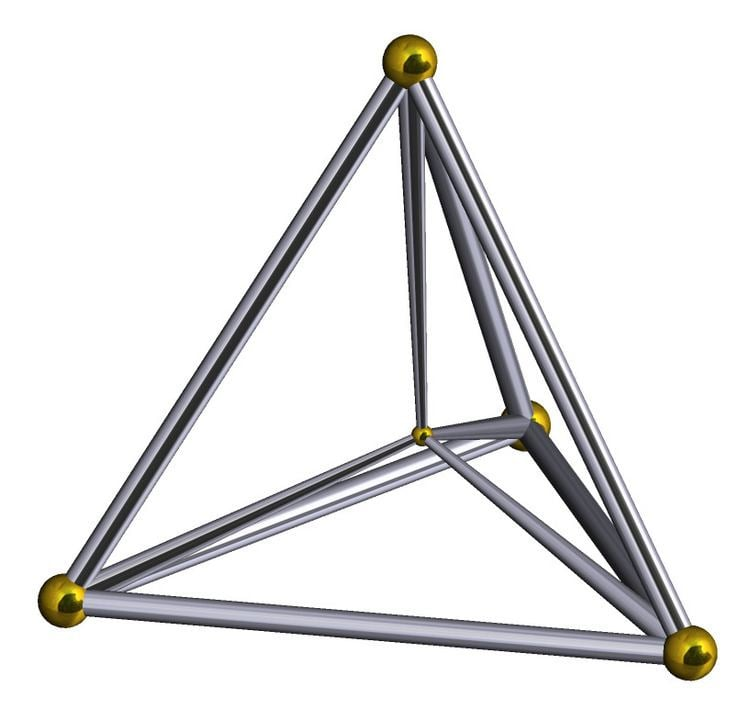# 5 cell

Updated on
Covid-19
 Schläfli symbol  {3,3,3}Faces  10 {3}Vertices  5 Cells  5 {3,3}Edges  10Type  Convex regular 4-polytope

In geometry, the 5-cell is a four-dimensional object bounded by 5 tetrahedral cells. It is also known as a C5, pentachoron, pentatope, pentahedroid, or tetrahedral pyramid. It is a 4-simplex, the simplest possible convex regular 4-polytope (four-dimensional analogue of a Platonic solid), and is analogous to the tetrahedron in three dimensions and the triangle in two dimensions. The pentachoron is a four dimensional pyramid with a tetrahedral base.

## Contents

The regular 5-cell is bounded by regular tetrahedra, and is one of the six regular convex 4-polytopes, represented by Schläfli symbol {3,3,3}.

## Alternative names

• Pentachoron
• 4-simplex
• Pentatope
• Pentahedroid (Henry Parker Manning)
• Pen (Jonathan Bowers: for pentachoron)
• Hyperpyramid, tetrahedral pyramid
• ## Geometry

The 5-cell is self-dual, and its vertex figure is a tetrahedron. Its maximal intersection with 3-dimensional space is the triangular prism. Its dihedral angle is cos−1(1/4), or approximately 75.52°.

## Construction

The 5-cell can be constructed from a tetrahedron by adding a 5th vertex such that it is equidistant from all the other vertices of the tetrahedron. (The 5-cell is essentially a 4-dimensional pyramid with a tetrahedral base.)

The simplest set of coordinates is: (2,0,0,0), (0,2,0,0), (0,0,2,0), (0,0,0,2), (τ,τ,τ,τ), with edge length 2√2, where τ is the golden ratio.

The Cartesian coordinates of the vertices of an origin-centered regular 5-cell having edge length 2 are:

( 1 10 ,   1 6 ,   1 3 ,   ± 1 ) ( 1 10 ,   1 6 ,   2 3 ,   0 ) ( 1 10 ,   3 2 ,   0 ,   0 ) ( 2 2 5 ,   0 ,   0 ,   0 )

Another set of origin-centered coordinates in 4-space can be seen as a hyperpyramid with a regular tetrahedral base in 3-space, with edge length 2√2:

( 1 , 1 , 1 , 1 / 5 ) ( 1 , 1 , 1 , 1 / 5 ) ( 1 , 1 , 1 , 1 / 5 ) ( 1 , 1 , 1 , 1 / 5 ) ( 0 , 0 , 0 , 5 1 / 5 )

The vertices of a 4-simplex (with edge √2) can be more simply constructed on a hyperplane in 5-space, as (distinct) permutations of (0,0,0,0,1) or (0,1,1,1,1); in these positions it is a facet of, respectively, the 5-orthoplex or the rectified penteract.

## Boerdijk–Coxeter helix

A 5-cell can be constructed as a Boerdijk–Coxeter helix of five chained tetrahedra, folded into a 4-dimensional ring. The 10 triangle faces can be seen in a 2D net within a triangular tiling, with 6 triangles around every vertex, although folding into 4-dimensions causes edges to coincide. The purple edges represent the Petrie polygon of the 5-cell.

## Projections

The A4 Coxeter plane projects the 5-cell into a regular pentagon and pentagram.

## Irregular 5-cell

There are many lower symmetry forms, including these found in uniform polytope vertex figures:

The tetrahedral pyramid is a special case of a 5-cell, a polyhedral pyramid, constructed as a regular tetrahedron base in a 3-space hyperplane, and an apex point above the hyperplane. The four sides of the pyramid are made of tetrahedron cells.

Many uniform 5-polytopes have tetrahedral pyramid vertex figures:

Other uniform 5-polytopes have irregular 5-cell vertex figures. The symmetry of a vertex figure of a uniform polytope is represented by removing the ringed nodes of the Coxeter diagram.

## Compound

The compound of two 5-cells in dual configurations can be seen in this A5 Coxeter plane projection, with a red and blue 5-cell vertices and edges. This compound has [[3,3,3]] symmetry, order 240. The intersection of these two 5-cells is a uniform birectified 5-cell. = .

## Related polytopes and honeycomb

The pentachoron (5-cell) is the simplest of 9 uniform polychora constructed from the [3,3,3] Coxeter group.

It is in the sequence of regular polychora: the tesseract {4,3,3}, 120-cell {5,3,3}, of Euclidean 4-space, and hexagonal tiling honeycomb {6,3,3} of hyperbolic space. All of these have a tetrahedral vertex figure.

It is similar to three regular polychora: the tesseract {4,3,3}, 600-cell {3,3,5} of Euclidean 4-space, and the order-6 tetrahedral honeycomb {3,3,6} of hyperbolic space. All of these have a tetrahedral cell.

## References

Similar Topics
Solo (2011 film)
Luke Allen Gale
Frank Pratt (politician)
Topics